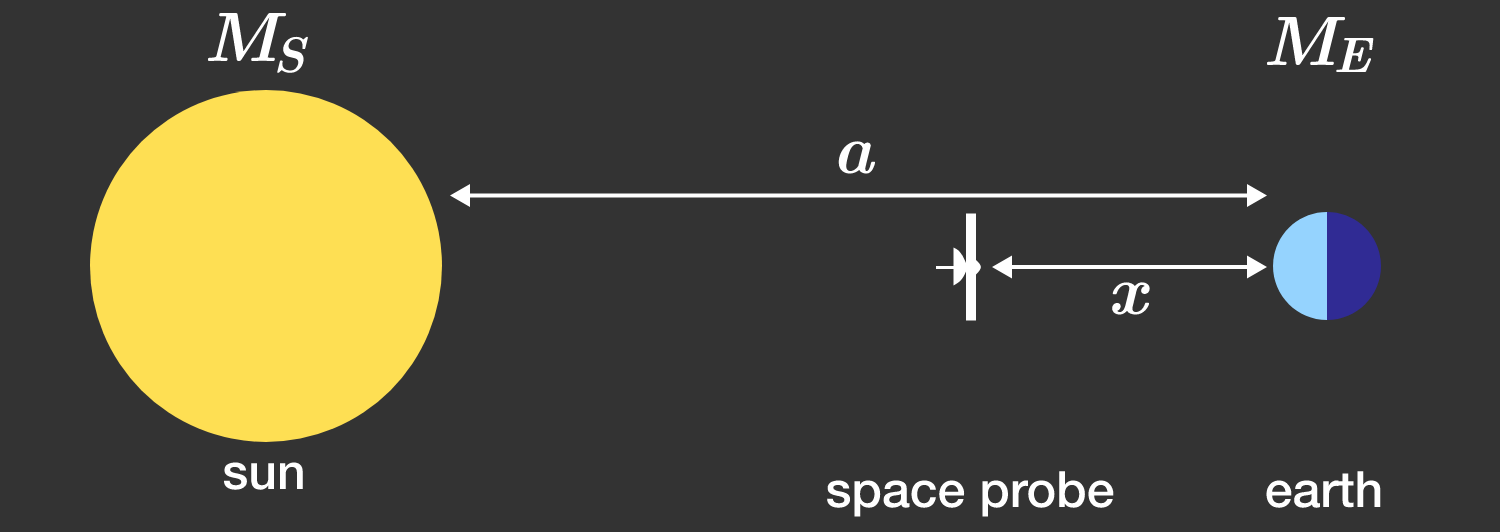# Stationary point between sun and earth

There is a special point between Sun and Earth where a spacecraft can be parked, so that it always remains directly between them, at a fixed distance $x$ from Earth, as Earth rotates around the Sun. A spacecraft can remain at this point without using any thrust.

What is the distance $x$ of this point from the Earth in gigameters? $\big(1\text{ gigameter}=10^6\text{ km}\big)$Details and Assumptions:

• The masses of the Earth and the Sun have a ratio of $1: 333,000.$
• The distance between the Earth and the Sun is $a \approx 150 \cdot 10^6 \text{ km}.$
• The radii of the Earth and the Sun are negligibly small.
×

Problem Loading...

Note Loading...

Set Loading...